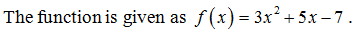# Given the function f(x)=3x^2+5x-7. evaluate the following .f(x+h)

Question
6 views

Given the function f(x)=3x^2+5x-7. evaluate the following .

f(x+h)

check_circle

Step 1...

### Want to see the full answer?

See Solution

#### Want to see this answer and more?

Solutions are written by subject experts who are available 24/7. Questions are typically answered within 1 hour.*

See Solution
*Response times may vary by subject and question.
Tagged in

### Math